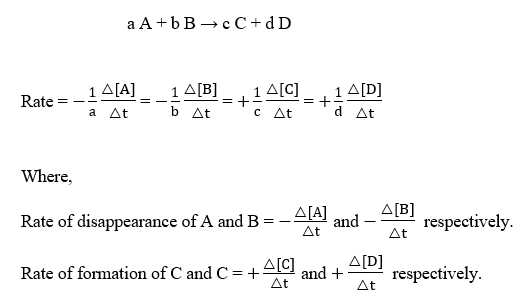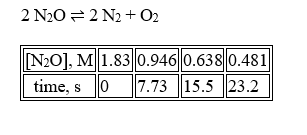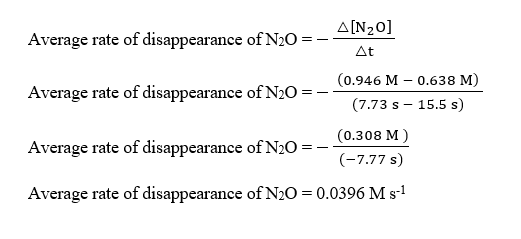# For the decomposition of nitrous oxide at 565 °C2 N2O2 N2 + O2the following data have been obtained:[N2O], M1.830.9460.6380.481  time, s07.7315.523.2The average rate of disappearance of N2O over the time period from t = 7.73 s to t = 15.5 s is  M s-1.

Question
1 views

For the decomposition of nitrous oxide at 565 °C

2 N2O2 N2 + O2

the following data have been obtained:

 [N2O], M 1.83 0.946 0.638 0.481 time, s 0 7.73 15.5 23.2

The average rate of disappearance of N2O over the time period from t = 7.73 s to t = 15.5 s is  M s-1.

check_circle

Step 1

The rate of a reaction is expressed as :Step 2

The given reaction and the data table are :At t = 7.73 s, [N2O] = 0.946 M

At t = 15.5 s, [N2O] = 0.638 M

Step 3

Therefore, the average rate of disappearance of N2O over the time period from t = 7.73 s to t = 15.5 s can be calculate as :...

### Want to see the full answer?

See Solution

#### Want to see this answer and more?

Solutions are written by subject experts who are available 24/7. Questions are typically answered within 1 hour.*

See Solution
*Response times may vary by subject and question.
Tagged in